# 计算机组成原理

## 计算机发展史（四个阶段）

### 第一阶段（1946~1957）：电子管计算机

世界上第一台计算机叫埃尼阿克(ENIAC)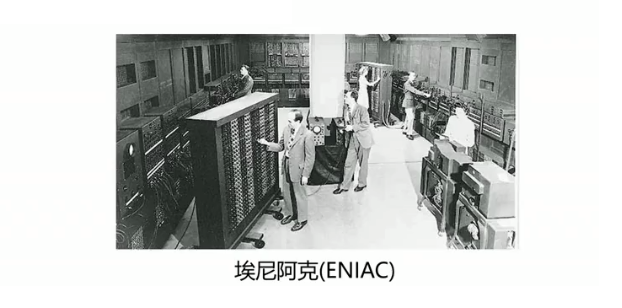第二次世界大战是电子管计算机产生的催化剂（英国为了解密德国海军的密文），在第二次世界大战中，战争使用了飞机和火箭，打得准需要计算设计参数，设计参数需要几千次运算才能计算出来，在没有计算机之前 ，这些都需要人工手动去计算，埃尼阿克的计算速度大约是手工计算的20万倍。

埃尼阿克(ENIAC)由18000多个电子管，运行耗电量达150千瓦，重量达30吨，占地1500平方英尺。由此可见，第一阶段的电子管计算机，集成度小，空间占用大，功耗高，运行速度慢，操作复杂，更换程序需要接线。

### 第二阶段（1957~1964）：晶体管计算机

贝尔实验室的三个科学家发明了晶体管，第一台晶体管计算机产生于麻省理工大学的林肯实验室。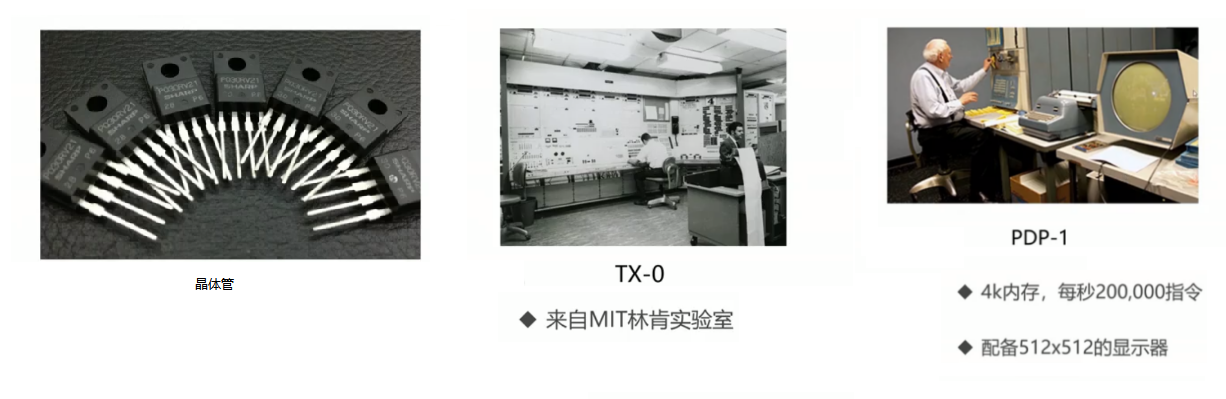### 第三阶段（1964~1980）：集成电路计算机

德州仪器的工程师发明了集成电路(IC)，后面就有了集成电路计算机，集成电路计算机让计算机具备进入千家万户的条件。因为集成电路使计算机变得更小，功耗变得更低，计算速度变得更快。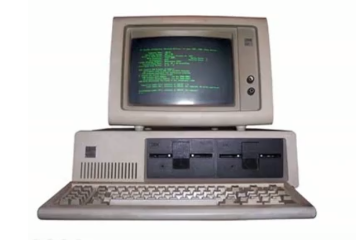关于集成电路计算机，IBM当时主要推出了两款计算机，使用量比较大，分别是7094和1401，但这两款计算机的主打功能不同，而且相互无法兼容，公司也不愿意投入两组人力，于时后来IBM推出了兼容的产品System/360，这也是操作系统的雏形。

### 第四阶段（1980~至今）：超大规模集成电路计算机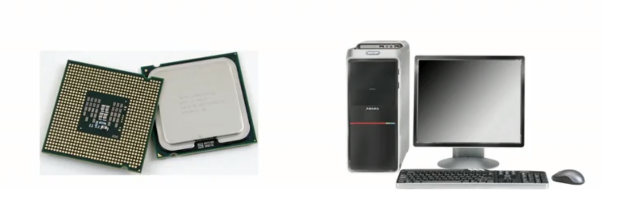超大规模集成电路，一个芯片上集成了上百万的晶体管，这样使超大规模集成电路计算机速度更快，价格更低，体积更小，更能被大众所接受，而且用途丰富，可以用作文本处理，表格处理，高交互的由于与应用等。

## 计算机的分类

### 超级计算机

功能最强、运算速度最快、存储容量最大的计算机，多用于国家高科技领域和尖端技术研究。

运算速度单位是TFlop/s，1TFlob/s=每秒一万亿次浮点数计算

### 大型计算机

大型计算机又称大型机、大型主机、主机等；具有高性能，可处理大数据与复杂的运算；在大型机市场领域，IBM占据着很大的份额。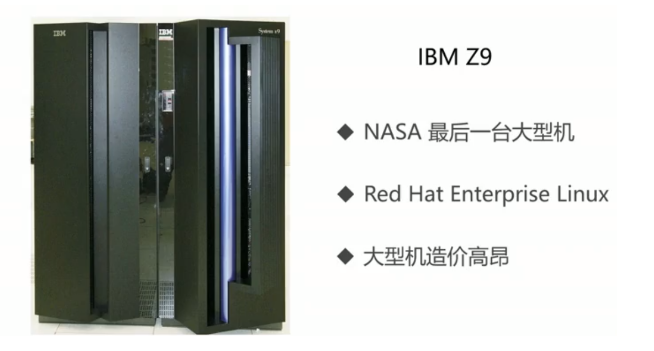去”IOE”行动，”IOE”是分别指IBM，Oracle，EMC，去”IOE”是阿里巴巴提出的概念，代表了高维护费用的存储系统，不够灵活，伸缩性弱。阿里巴巴在2008年提出去“IOE”行动，在2009年成立了阿里云。

### 迷你计算机（服务器）

迷你计算机也称为小型机，即普通服务器，不需要特殊的空调场所，具备不错的算力，可以完成较复杂的运算。普通的服务器已经替代了传统的大型机，成为大规模企业计算的中枢。

### 工作站

高端的通用微型计算机，提供比个人计算机更强大的性能，类似于普通台式电脑，体积较大，但性能较强。### 微型计算机

微型计算机又称为个人计算机，是最普通的一类计算机。麻雀虽小，五脏俱全，从构成的体质上来讲，个人计算机与前面的分类的无异。## 计算机的体系与结构

### 冯诺依曼体系

冯诺依曼体系：将程序指令和数据一起存储的计算机设计概念结构。在冯诺依曼体系出现前，早期的计算机仅含固定用途程序，如改变程序就需要更改结构，重新设计电路，也就是不能先打会儿游戏然后再写会儿代码，这样就很影响我们的使用体验，所以就提出将程序存储起来，并设计成通用电路，即存储程序指令，设计通用电路。

冯诺依曼体系提出：计算机必须有一个存储器、有一个控制器、有一个计算器、有输入设备和输出设备。这样就能够把需要的程序和数据送至计算机中（输入设备），能够长期记忆程序、数据、中间结果及最终运算结果的能力（存储器），能够具备算术、逻辑运算和数据传送等数据加工处理的能力（运算器、控制器），能够按照要求将处理结果输出给用户（输出设备）。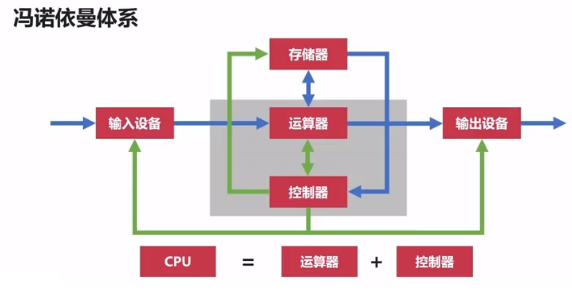由于CPU处理数据的速度要远快于存储器的存储速度，所以CPU和存储器的分开会造成芬诺伊曼瓶颈，也就是CPU和存储器之间的速率问题无法调和。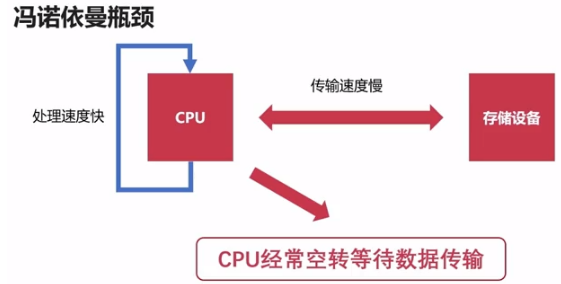### 现代计算机的结构

现代计算机在冯诺曼体系结构基础上进行修改，解决CPU与存储设备之间的性能差异问题。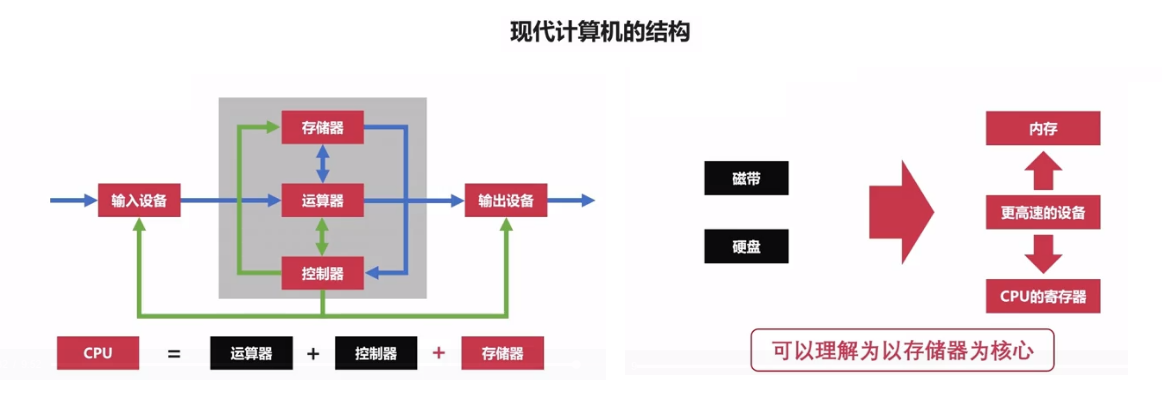## 计算机的层次与编程语言

### 程序翻译与程序解释

我们将人类语言变为计算机能看懂的语言，需要进行语言之间的转换。程序翻译就是将较为高级的计算机语言L1转为较为低级的计算机语言L0（编译器），L0就是计算机实际执行的语言。程序解释就是把较为高级的语言L1，作为用L0语言实现的一个程序的输入，然后得到较为低级计算机语言L0。

• 计算机实际执行的指令都是L0
• 翻译过程生成新的L0程序，解释过程不生成新的L0程序
• 解释过程由L0编写解释器去执行L1程序

程序翻译的常见语言：C/C++, Object-C, Golang; 程序解释的常见语言：Python, Php, JavaScript。而Java和C#是属于翻译加解释性语言，Java程序转为JVM字节码的过程是程序翻译，由JVM字节码变成机器码的过程是程序解释。

### 计算机的层次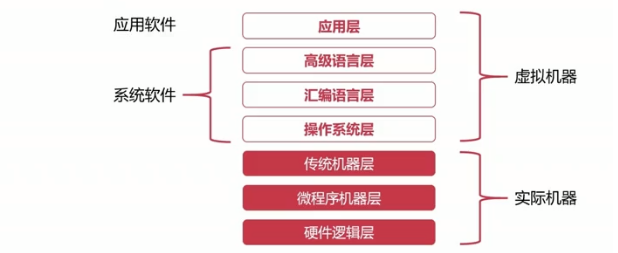## 计算机的字符与编码集

使用7个bits就可以完全表示ASCII码，包含95个可打印字符，33个不可打印字符（包括控制字符），即 33+95=128=27，但是这个ASCII码在很多应用和很多国家中的符号都无法表示，例如数学符号：“$$\div$$ $$\neq$$ $$\geq$$ $$\approx$$ $$\pi$$”。所以在第一次对ASCII码进行扩充时，7bits=> 8bits，在可拓展的ASCII码中，包含了常见的运算符，带音标的欧洲字符，和其他常用符、表格等。

字符编码集的国际化：由于欧洲、中亚、东亚、拉丁美洲国家的语言多样性，造成语言体系不一样，不以有限字符组合的语言，尤其是中国、韩国、日本的语言最为复杂。国标2312（GB2312）：《信息交换用汉字编码字符集-基本集》是中文编码集，一共收录了7445个字符，包括6763个汉字和682个其他符号。Unicode(统一码、万国码、单一码)定义了世界通用的符号集，UTF-*实现了编码，UTF-8以字节为单位对Unicode进行编码。Unicode是兼容全球的字符集。

## 计算机的总线与IO设备

### 计算机的总线

总线提供了对外的接口，不同设备可以通过USB（Universal Serial Bus）接口进行连接，连接的标准，促使外围设备接口的统一。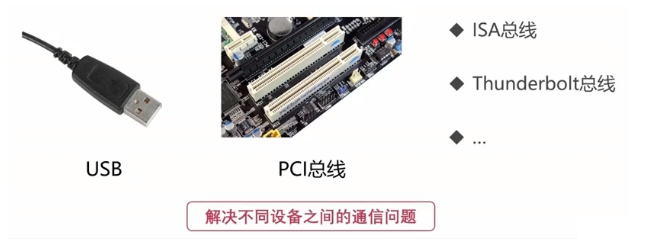当我们的输入设备向计算机中输入信息，要求计算机给出指定输出时，这时需要IO总线来进行总线连接。

• 片内总线：芯片内部的总线，寄存器与寄存器之间，寄存器与控制器、运算器之间。片内总线是高集成度芯片内部的信息传输线。
• 系统总线：CPU、主内存、IO设备、各组件之间的信息传输线
• 数据总线：双向传输各个部件的数据信息，数据总线的位数（总线宽度）是数据总线的重要参数，一般与CPU位数相同（32位、64位）
• 地址总线：指定源数据或目的数据在内存中的地址，地址总线的位数与存储单元有关。若地址总线位数=n，寻址范围：0~2n
• 控制总线：控制总线是用来发出各种控制信号的传输线，控制信号经由控制总线从一个组件发给另外一个组件，控制总线可以监视不同组件之间的状态（就绪/未就绪）

• 链式查询：好处：电路复杂度低，仲裁方式简单；坏处：优先级低的设备难以获得总线的使用权，对电路故障敏感。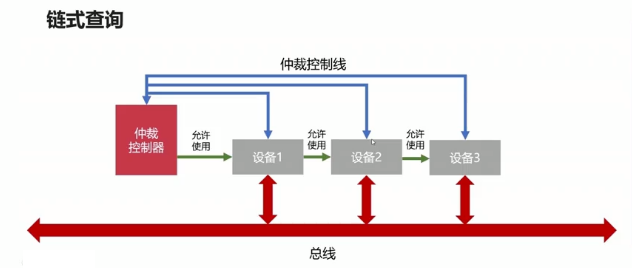• 计时器定时查询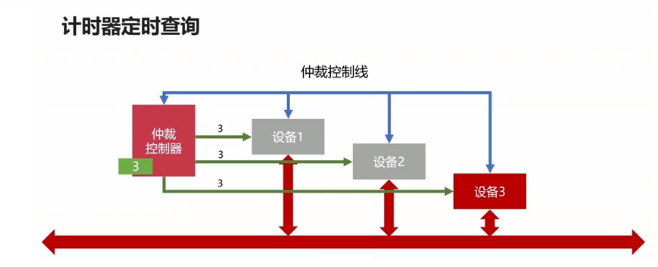• 独立请求
• 每个设备均有总线独立连接仲裁器
• 设备可单独向仲裁器发送请求和接受请求
• 当同时收到多个请求信号，仲裁器有权按优先级分配使用权
• 好处：响应速度快，优先顺序可动态改变，设备连线多，总线控制复杂。

### 常见的IO设备

常见的输入设备：分为字符输入设备和图像输入设备。字符输入设备就如键盘，图像输入设备如鼠标、数位板、扫描仪等。常见的输出设备：显示器、打印机、投影仪

输入输出接口的通用设计：我们需要考虑如何向设备发送数据？如何读取数据？该设备有没被占用？设备是否已经启动？设备是否已经连接？等等还有其他问题，基于对输入输出设备接口通用设计的考虑，至少应该有以下几部分：

• 数据线
• 是I/O设备与主机之间进行数据交换的传送线
• 单向传输数据线
• 双向传输数据线
• 状态线
• IO设备状态向主机报告的信号线
• 查询设备是否已经正常连接并就绪
• 查询设备是否已经被占用
• 命令线
• CPU向设备发送命令的信号线
• 发送读写信号
• 发送启动停止信号
• 设备选择线
• 主机选择I/O设备进行操作的信号线
• 对连在总线上的设备进行选择

## 计算机的存储器

• 按存储介质分：
• 半导体存储器：例如内存、U盘、固态硬盘
• 磁存储器：例如磁带、磁盘等
• 按存取方式分类：
• 随机存储器（RAM）：随机读取，与位置无关
• 串行存储器：与位置有关，按顺序查找
• 只读存储器（ROM）：只读不写，BIOS就是存储在只读存储器中

对于存储器，我们希望它读写速度快，存储容量大，而价格最好低一些。存储器的层次结构如下图：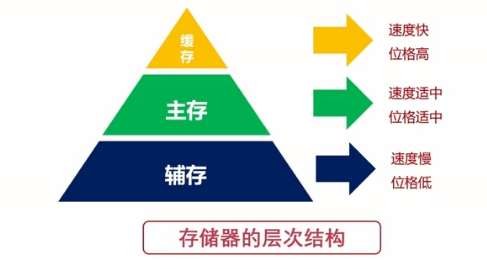缓存：指的就是CPU中的寄存器以及高速缓存；主存：计算机中的内存；辅存：计算机的外部存储设备，比如磁盘、U盘、移动硬盘等。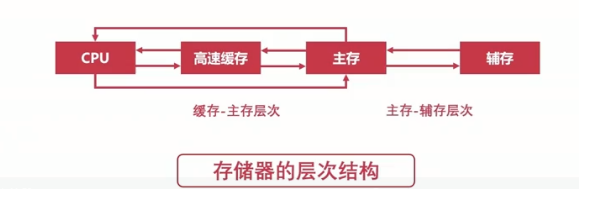• 原理：局部性原理，局部性原理就是指CPU访问存储器时，无论是存取指令还是存取数据，所访问的存储单元都趋集于在一个较小的连续区域中。
• 实现：在CPU与主存之间增加一层速度快（容量小）的Cache
• 目的：解决主存速度不足的问题

• 原理：局部性原理
• 实现：主存之外增加辅助存储器（磁盘，SD卡，U盘等）
• 目的：解决主存容量不足的问题

计算机突然断电，内存数据就会丢失，但是计算机断电，磁盘数据不会丢失。

• 主存储器——内存
• RAM（随机存取存储器 ：Random Access Memory）
• RAM通过电容存储数据，必须隔一段时间刷新一次
• 如果断电，那么一段时间后将丢失所有数据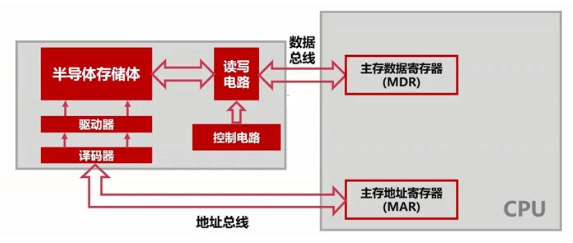对于32位系统，计算机内存最大为 232 = 430 = 4GB，对于64为系统，计算机内存最大为 264 = 234 x 230 = 234GB
• 辅助存储器——磁盘
• 表面是可磁化的硬磁特性材料
• 移动磁头径向运动读取磁道信息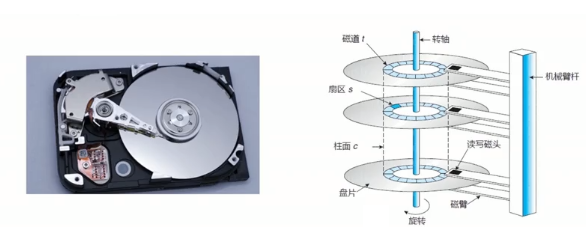计算机的辅助存储器常用算法
• 先来先服务算法
• 最短寻道时间优先
• 与磁头当前位置有关
• 优先访问离磁头最近的磁道
• 扫描算法（电梯算法）
• 循环扫描算法

## 计算机的高速缓存

### 高速缓存的工作原理

：是指存放在一个存储单元中的二进制代码组合
字块：存储在连续的存储单元中而被看作是一个单元的一组字节
一个字快有32位，一个字快共B个字，主存中共M个字块，则主存总字数=B*M，主存总容量(bits)=B*M*32。字的地址包含两部分：前m位指定字快的地址，后b位指定字在字快中的地址。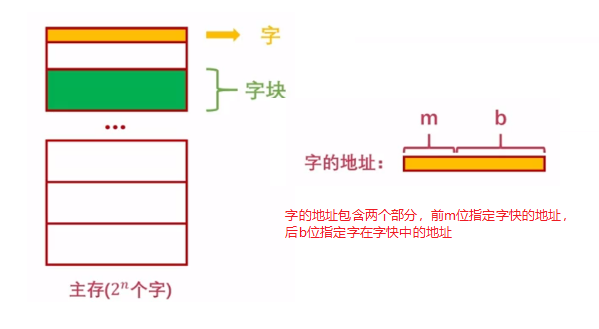由于1G=1024M，所以4G=4096M，由于字快大小为4M，所以字快数为：$$4096\div4=1024$$，所以字快地址m=$$\log_2 1024=10$$，块内字数为$$4M\div32bit=1048576bit$$，块内地址b=$$\log_2 1048576=20$$，所以快地址m的位数至少为10，块内地址b的位数最少为20。

我们已经知道在CPU与主存之间存在高速缓存，当CPU需要的数据在缓存里时，则CPU直接从高度缓存中读取数据即可，不需要去访问主存，但当CPU需要的数据不在缓存里时，则需要去主存拿。由于CPU的处理速度远远快于主存的读取速度和数据传输速度，所以这样会导致CPU空转来等待数据传输，造成资源上的浪费。所以我们尽可能的让CPU去高速缓存中读取数据，因此我们需要一个量化指标，这个量化指标就是命中率。命中率是衡量缓存的重要性能指标，理论上CPU每次都能从高速缓存中读取数据的时候，命中率为1。假设访问主存次数为$$N_m$$，访问Cache次数为：$$N_c$$，则命中率h为：$$h=\frac{N_c}{N_c+N_m}$$。现在来看看访问效率e，假设访问主存时间为$$t_m$$，访问缓存时间为$$t_c$$，则访问Cache-主存系统的平均时间为：$$t_a=ht_c+(1-h)t_m$$，则访问效率e为：$$e=\frac{t_c}{t_a}=\frac{t_c}{ht_c+(1-h)t_m}$$

命中率：$$h=\frac{N_c}{N_c+N_m}=\frac{2000}{2000+50}=0.97$$

访问效率：$$e=\frac{t_c}{t_a}=\frac{t_c}{ht_c+(1-h)t_m}=\frac{50}{0.97\ast50+(1-0.97)200}=0.917=91.7\%$$

平均访问时间：$$0.97\ast50+(1-0.97)200=54.5ns$$

### 高速缓存的替换策略

由于高速缓存的运行需要良好的缓存替换策略。什么是缓存替换策略呢？就是当高速缓存中没有数据时，需要从主存中载入所需要的数据。高速缓存中常见的替换策略：

• 随机算法
• 先进先出算法（FIFO）
• 最不经常使用算法（LFU）
• 优先淘汰最不经常使用的字快
• 需要额外的空间记录字快的使用频率
• 最近最少使用算法（LRU）
• 优先淘汰一段时间内没有使用的字快
• 有多种实现方法，一般使用双向链表
• 把当前访问节点置于链表前面（保证链表头部节点是经常使用的）

## 计算机的指令系统

1. 机器指令的形式
机器指令主要有两部分组成：操作码、地址码。地址码直接给出操作数和操作数的地址，分三地址指令、二地址指令和一地址指令，最后还有零地址指令，零地址指令在机器指令中没有地址码，用来进行空操作、停机操作、中断返回操作等。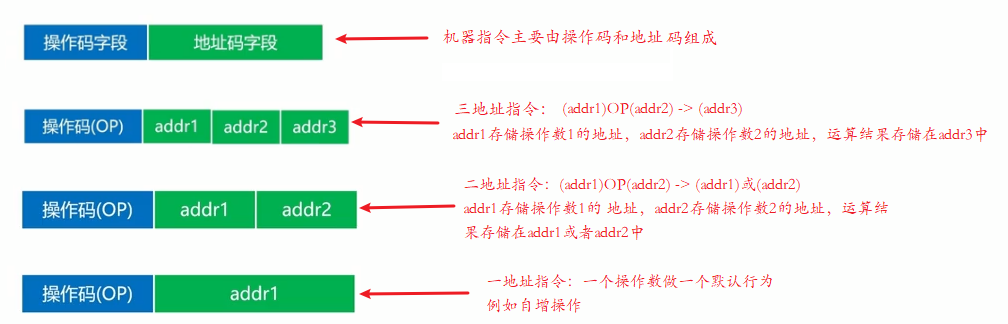2. 机器指令的操作类型：
• 数据传输：在寄存器之间、寄存器与存储单元、存储单元之间传送；还可以进行数据独写、交换地址数据、清零置一等操作。
• 算术逻辑操作：操作数之间的加减乘除运算；操作数的与或非等逻辑运算
• 移位操作：数据左移（乘2）、数据右移（除2）；完成数据在算术逻辑单元的必要操作。
• 控制指令：主要有等待指令、停机指令、空操作指令、中断指令等。
3. 机器指令的寻址方式
• 指令寻址：顺序寻址；跳跃寻址
• 数据寻址：
• 立即寻址：指令直接获得操作数，无需访问存储器
• 直接寻址：直接给出操作数在主存中的地址，寻找操作数简单，无需计算数据地址
• 间接寻址：指令地址码给出的是操作数地址的地址，需要访问一次或多次主存来获取操作数

## 计算机的控制器

• 程序计数器：程序计数器用来存储下一条指令的地址；循环从程序计数器中拿出指令；当指令被拿出时，指向下一条指令
• 时序发生器：电气工程领域，用于发送时序脉冲；CPU根据不同的时序脉冲有节奏的进行工作
• 指令译码器：指令译码器是控制器主要部件之一，计算机指令由操作码和地址码组成，翻译操作码对应的操作以及控制传输地址码对应的数据
• 各种寄存器
• 指令寄存器：指令寄存器也是控制器的主要部件之一，从主存或高速缓存读取计算机指令
• 主存地址寄存器：保存当前CPU正要访问的内存单元的地址
• 主存数据寄存器：保存当前CPU正要读或写的内存数据
• 通用寄存器：用于暂时存放或传送数据和指令，可保存ALU的运算中间结果，容量比一般的专用寄存器要大
• 总线

## 计算机的运算器

• 数据缓冲器：分为输入缓冲和输出缓冲，输入缓冲暂时存放外设送过来的数据；输出缓冲暂时存放送往外设的数据
• ALU：算术逻辑单元，是运算器的主要组成，常见的位运算（左右移、与或非等），算数运算（加减乘除等）
• 通用寄存器
• 状态字寄存器：存放运算状态（条件码、进位、溢出、结果正负等）；存放运算控制信息（调试跟踪标记位、允许中断位等）
• 总线

## 计算篇

### 进制概述

进制是一种计数方式，亦称为进位计数法或位值计数法，用有限种数字符号来表示无线的数值，使用的数字符号的数目称为这种进位制的基数或底数。例如n=10[0-9]称为十进制；还有例如玛雅文明的玛雅数字，因努伊特的因努伊特数字使用的就是二十进制；像时间、坐标、角度等量化数据使用的就是六十进制。但我们使用的计算机喜欢二进制，但是使用二进制表达太长了，使用大进制可以解决这个问题，计算机常用的大进制有八进制、十六进制。因为八进制和十六进制都满足2的n次方要求。例如1024分别使用二进制、八进制、十六进制表示为：1024 = 0b1000000000 = oO2000 = ox400

### 二进制的运算基础

1.整数十进制和二进制的互相转换
(整数)十进制转换二进制：重复相除法。例子：十进制数101转为二进制：如下

101/2 50 1
50/2 25 0
25/2 12 1
12/2 6 0
6/2 3 0
3/2 1 1
1/2 0 1

最后，倒着取余数，所以101的二进制表示是：1100101。二进制转为十进制使用的是按权展开法：$$1100101 = 1 \times 2^6 + 1 \times 2^5 + 0 \times 2^4 + 0 \times 2^3 + 1 \times 2^2 +0 \times 2^1 + 1 \times 2^0 = 101$$
再举一个例子：十进制数237转为二进制，如下

237/2 118 1
118/2 59 0
59/2 29 1
29/2 14 1
14/2 7 0
7/2 3 1
3/2 1 1
1/2 0 1

最后倒着取数，所以237的二进制表示是：11101101。使用按权展开法把二进制转为十进制：$$11101101 = 1 \times 2^7 + 1 \times 2^6 + 1 \times 2^5 + 0 \times 2^4 + 1 \times 2^3 + 1 \times 2^2 +0 \times 2^1 + 1 \times 2^0 = 237$$
二进制转为十进制：按权展开法的的计算公式如下：
$$N = d_{n-1}d_{n-2}…d_1d_0 = d_{n-1}r^{n-1} + d_{n-2}r^{n-2} + d_1r +d_0$$

2.小数十进制和二进制的互相转换
如果这个十进制是小数，则使用重复相乘法。例如 $$\frac{25}{32}$$转为二进制，如下：

$$\frac{25}{32} \times 2$$ $$\frac{25}{16} = 1+\frac{9}{16}$$ 1
$$\frac{9}{16} \times 2$$ $$\frac{9}{8} = 1+\frac{1}{8}$$ 1
$$\frac{1}{8} \times 2$$ $$\frac{1}{4} = 0+\frac{1}{4}$$ 0
$$\frac{1}{4} \times 2$$ $$\frac{1}{2} = 0+\frac{1}{2}$$ 0
$$\frac{1}{2} \times 2$$ $$1 = 1+0$$ 1

最后顺着取整数：$$\frac{25}{32}$$的二进制表示为：0.11001。现在再使用按权展开法把二进制转为十进制数：
$$N = 0.11001 = 1 \times 2^{-1} + 1 \times 2^{-2} + 0 \times 2^{-3} + 0 \times 2^{-4} + 1 \times 2^{-5} = 0.78125 = \frac{25}{32}$$

### 有符号数和无符号数

正的237使用二进制表示为：+237 = 011101101 ，负的237使用二进制表示为：-237 = 111101101，在计算机中，使用0表示正数，使用1表示负数。但计算机是怎么判断它是数字位还是符号位的呢？这就需要使用原码表示法了，在原码表示法中，使用0表示正数，使用1表示负数，规定符号位位于数值的第一位，表达简单明了，是我们最容易理解的表示法。0有两种表示法：00或10，在使用原码进行运算时，会非常复杂，特别是两个操作符号不同的时候，我们需要进行判断两个操作数得绝对值大小，使用绝对值大的数减去绝对值小的数，对于符号值，以绝对值大的为准。因此我们希望找不到不同符号操作数运算更加简单得方法，也就是可以使用正数来代替负数，使用加法操作来代替减法操作，从而消除减法。于是出现了二进制的补码表示法，补码的定义如下：

$x = \begin{cases} x & 2^{n} > x \geq 0 \\ 2^{n+1} + x & 0 > x \geq -2^n \end{cases}$

我们引入补码的初衷是由于减法运算复杂，希望找到使用正数代替负数的方法，使用加法代替减法操作，从而消除减法，但是通过上面例子可以看到，补码的引入，并没有实现校除减法的目的。于是就提出了反码，反码的目的是找出原码和补码之间的规律，消除转换过程中的减法，反码的定义如下：

$x = \begin{cases} x & 2^{n} > x \geq 0 \\ (2^{n+1} – 1) + x & 0 > x \geq -2^n \end{cases}$

13 0,1101 0,1101 0,1101
-13 1,1101 1,0011 1,0010
-7 1,0111 1,1001 1,1000
-1 1,0001 1,1111 1,1110

根据这个表格中数据，我们可以发现正数原码、补码、反码的值都是一样的；负数的反码等于原码除符号位外都按位取反，负数的补码等于反码+1。同样的，小数的二进制也满足这个规律，二进制小数的补码定义如下：

$x = \begin{cases} x & 1 > x \geq 0 \\ 2 + x & 0 > x \geq -1 \end{cases}$

### 定点数与浮点数

定点数的表示方法：纯小数的小数点放在符号位与数值位之间，纯整数的小数点放在数值位末尾，我们的定点数的保存需要乘以比例因子。

0.1011 0 1011
-0.1011 1 1011

1011 0 1011
-1011 1 1011

浮点数的表示格式：使用科学计数法表示浮点数，$$123450000000 = 1.2345 \times 10^11$$，1.2345是尾数，10是基数，11是阶码。浮点数的表示格式为：$$N = S \times r^j$$，S代表尾数，r代表基数，j表示阶码，尾数规定使用纯小数。例子如下：
$$N = S \times r^j$$
$$11.0101 = 0.110101 \times 2^10$$，这里都是二进制表示，阶码10对应十进制数为2
$$11.0101 = 0.0110101 \times 2^11$$，这里都是二进制表示，阶码11对应十进制数为3

0 10 0 11010100
0 11 0 01101010

浮点数的表示范围：假设阶码值取m位，尾数值取n位，$$N = S \times r^j$$，阶码能够表示的最大值为：$$2^m-1$$，阶码表示范围为：$$[-(2^m-1),2^m-1]$$；尾数能够表示的最大值为：$$1 – 2^{-n}$$，尾数能够表示的最小值为：$$2^{-n}$$，尾数表示的范围为：$$[-(1 – 2^{-n}),-2^{-n}]$$  $$[2^{-n},1-2^{-n}]$$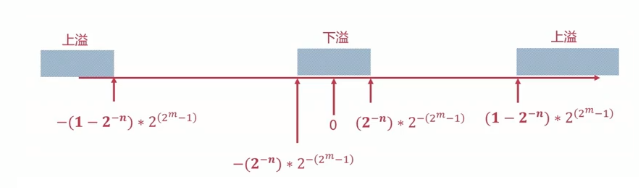由于正数的原码=反码=补码：$$x = 0.0001101000$$，浮点数的规格化：$$x = 0.1101000 * 2^{-11}$$

1 0011 0 1101000000

原码：$$x = 1,110110$$，浮点数的规格化：$$x = -0.110110 * 2^{-110}$$，由于尾数为110110 0000，则尾数反码为：001001 1111，尾数补码为：001010 0000

0 0110 1 001010 0000

• 定点数与浮点数位数相同时，浮点数表示的范围更大
• 当浮点数尾数为规格化数时，浮点数的精度更高
• 浮点数运算包含阶码和位数，浮点数的运算更为复杂

### 定点数的加减法运算

A[补] = 1,001110，B[补] = B[原] = 0,001101，A[补] + B[补] = (A+B)[补] = 1,011011，则A + B = -100101

A[补] = 1,1.0101110，B[补] = B[原] = 0.0110100，A[补] + B[补] = (A+B)[补] = 1,1.1100010，则A + B = -0.0011110

A[补] = 1,01110000，B[补] = 1,00110000，A[补] + B[补] = (A+B)[补] = 0,10100000，则A + B = 10100000，将结果转为十进制表示是160。我们可以把A和B转为十进制进行运算：A=-144，B=-208，A+B=-352，我们会发现二进制的计算结果是错误的。这是由于我们在计算时，对符号位的进位自然丢掉了，这是就发生了溢出，那我们如何来判断溢出呢？双符号位判断法：单符号位表示变成双符号位：0=>00，1=>11，双符号位产生的进位丢弃，结果的双符号位不同则表示溢出。

-B[补]等于B[补]连同符号位按位取反，末位加一

### 浮点数的加减法运算

运算过程：对阶->尾数求和->尾数规格化->舍入->溢出判断
$$x = 0.1101 \times 2^{01}$$
$$y = (-0.1010) \times s^{11}$$

00 0001 00 1101
00 0011 11 1010

$$x = 0.001101 \times 2^{11}$$
$$y = (-0.1010) \times s^{11}$$

00 0011 00 0011(01)
00 0011 11 1010

00 0011 11 1001

00 0011 11 1001

S = (x + y)[补] = 11.1001 = 11.(1)0010